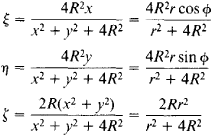Riemann Sphere

Also found in: Wikipedia.

Riemann sphere

[′rē‚män ‚sfir]
(mathematics)
The two-sphere whose points are identified with all complex numbers by a stereographic projection. Also known as complex sphere.

Riemann Sphere

a geometric representation introduced by B. Riemann for the set of complex numbers. The complex number

z = x + iy = r(cos ɸ + i sin ɸ) = re

can be represented by the point in the complex plane with the Cartesian coordinates x,y or the polar coordinates r, ɸ. To construct a Riemann sphere, a sphere is placed so as to be tangent to the complex plane at the origin of coordinates, and the points of the plane are mapped onto the surface of the sphere by means of a stereographic projection.

Each complex number is thus represented by a corresponding point of the sphere, which is called a Riemann sphere. The number 0 here is represented by the south pole of the Riemann sphere. Numbers with the same argument ɸ = constant (rays in the complex plane) are represented on the sphere by meridians. Numbers with the same modulus r = constant (circles in the complex plane) are represented by parallels of the Riemann sphere. No point of the complex plane corresponds to the north pole of the Riemann sphere. To preserve the one-to-one correspondence between points of the complex plane and the Riemann sphere, there is adjoined to the plane a “point at infinity,” which is assumed to correspond to the north pole and designated by z = ∞. Thus, the complex plane contains a single point at infinity, in contrast to a projective plane.

Suppose a system of rectangular Cartesian coordinates ξ, n, ζ is introduced in such a way that the ξ-axis and η-axis coincide with the x-axis and y-axis, respectively. There then corresponds to the point x + iy of the complex plane the pointof the Riemann sphere. The equation of the sphere is ξ2 + η2 + ζ2 - 2Rζ = 0.

Mentioned in ?
References in periodicals archive ?
The paper is an interval dynamics counterpart of three theories founded earlier by the authors in the setting of the iteration of rational maps on the Riemann sphere: the equivalence of several notions of non-uniform hyperbolicity, geometric pressure, and nice inducing schemes methods leading to results in thermodynamical formalism.
As can be seen from Figure 1, the shortest arc length on the circle is obtained from a plane-cut Riemann sphere determined by 3 points of the center of the ball, [phi]([c.sub.1]) and [phi]([c.sub.2]).
Project the original data onto the Riemann sphere and calculate the gap metric for each variable, and the matrix X* is calculated:
The Enriched Riemann Sphere is [CP.sup.1.sub.*] := {[[alpha].sub.*]}.
Paunescu, Enriched Riemann sphere, Morse stability and equi-singularity in [O.sub.2], Jour.
For a continuous space one can map the complex plane C = [R.sup.2] and unitary plane [C.sup.2] = [R.sup.4] to the 2-D Riemann sphere. Its 2-D surface has no demarcations, thus allowing any small or large rotation.
There is also a conjugate plane [C'.sup.2] for the antiparticles and its Riemann sphere. The conjugate quaternion is q' = w1 - xi - yj - zk.
Dynamics on the Riemann sphere; a Bodil Branner festschrift.
Then we deal with functions on the Riemann sphere [S.sup.2], which, as is well known, can be identified to the complex projective plane [P.sup.2](C); at least this was known to me at the time .
Let [{[D.sub.n]}.sup.[infinity].sub.n=1] be a sequence of domains in the Riemann sphere such that [z.sub.0] [member of] [D.sub.n], for some fixed point [z.sub.0] in the Riemann sphere.
The final two sections cover integrable structures in topological field theories, including the explicit quantum ordering for a special class of quantum geodesic functions, discrete analogues of the Dirac magnetic monopole, and the twistor description of harmonic maps of the Riemann sphere, and algebraic methods in topological field theory and the theory of integrable systems, with discussion of algebras of conjugacy classes of partial elements and Lax operator algebras of type G2.
e., the famous Riemann sphere formed by augmenting the complex plane C by the "point at infinity".

Site: Follow: Share:
Open / Close